StatLect

# Probability density function

The probability density function (pdf) is a function that completely characterizes the distribution of a continuous random variable.## Explanation

There are two main ways to specify the probability distribution of a random variable:

1. assign a probability to each value that the variable can take;

2. assign probabilities to intervals of values that the variable can take.

Method 1 is used when the set of possible values of the variable is countable (the variable is discrete).

Method 2 is applied if the set is uncountable (the variable is continuous) and Method 1 cannot be used. Method 2 involves the probability density function. Let us see why and how.

## The problem of continuous variables

Method 1 cannot be employed when the set of possible values is uncountable.

This impossibility is due to a number of fundamental mathematical reasons. For example:

• the elements of an uncountable sets cannot be arranged into a sequence; if we attempted to assign probabilities to each possible value of the variable, we would have no order to follow;

• even if we managed to assign the probabilities to the single values, we would not be able to check that their sum is equal to 1; in fact, there is no way of summing all the numbers in an uncountable set.

To circumvent this impossibility, mathematicians invented a "trick" that relies on probability density functions and integrals.

## How the pdf works

The probability that a continuous random variable takes a value in a given interval is equal to the integral of its probability density function over that interval.

In turn, the integral is equal to the area of the region in the xy-plane bounded by:

• the x-axis;

• the pdf;

• the vertical lines corresponding to the boundaries of the interval.

For example, in the picture below the blue line is the pdf of a normal random variable, and the area of the grey region is equal to the probability that the random variable takes a value in the interval between -2 and 2.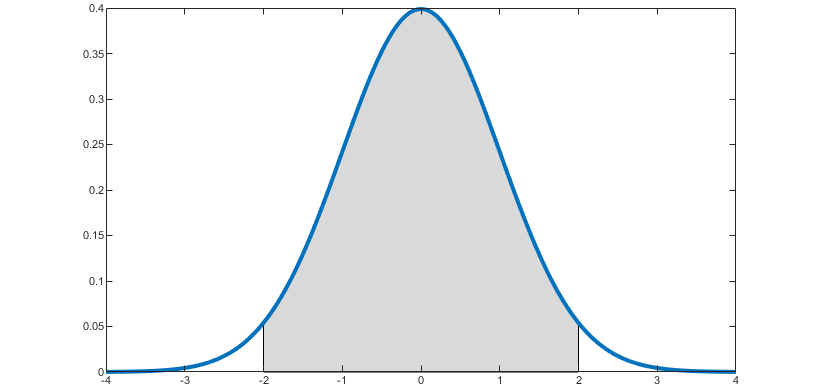## Definition

The following is a formal definition.

Definition The probability density function of a continuous random variableis a functionsuch that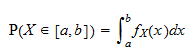for any interval.

The set of valuesfor whichis called the support of.

## Example

Suppose that a random variablehas probability density function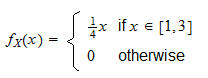In order to compute the probability thattakes a value in the interval, we need to integrate the probability density function over that interval: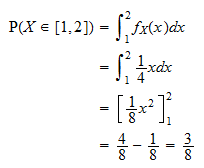## The probability density is not a probability

It is important to understand a fundamental difference between:

• the probability density function, which characterizes the distribution of a continuous random variable;

• the probability mass function, which characterizes the distribution of a discrete random variable.

Remember that:

• a discrete random variable can take a countable number of values;

• a continuous random variable can take an uncountable number of values.

The probability mass function of a discrete variableis a functionthat gives you, for any real number, the probability thatwill be equal to.

On the contrary, ifis a continuous variable, its probability density functionevaluated at a given pointis not the probability thatwill be equal to. As a matter of fact, this probability is equal to zero for anybecause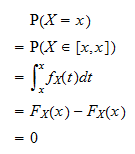whereis any primitive (or indefinite integral) of.

## Interpretation of the pdf

Although it is not a probability, the value of the pdf at a given pointcan be given a straightforward interpretation: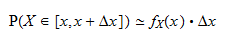whereis a small increment.

Proof

The proof we are going to give is not rigorous. Rather, we are focusing on the intuition. For the sake of simplicity, we assume that the pdf is a continuous function. Strictly speaking, this is not necessary, although most of the pdfs that are encountered in practice are continuous (by definition, a pdf must be integrable; however, while all continuous functions are integrable, not all integrable functions are continuous). If the pdf is continuous andis small, thenis well approximated byfor anybelonging to the interval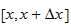. It follows that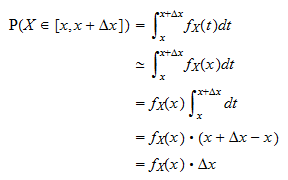In the above approximate equality, we consider the probability thatwill be equal toor to a value belonging to a small interval near. In particular, we consider the interval.

The probability is proportional to the lengthof the small interval we are considering.

The constant of proportionalityis the probability density function ofevaluated at.

Thus, the higher the pdfis at a given point, the higher is the probability thatwill take a value near.

## Related concepts

Related concepts are those of:

## Properties

The properties that a pdf needs to satisfy are discussed in the lecture on legitimate probability density functions.

## More details, examples and solved exercises

More details about the pdf, examples and solved exercises can be found in the lecture on Random variables.

Previous entry: Prior probability

Next entry: Probability mass function